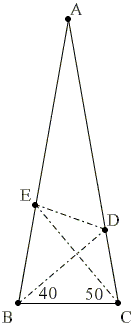## The 80-80-20 Triangle Problem, 40-50 Variant, Solution #1Let ABC be an isosceles triangle (AB = AC) with ∠BAC = 20°. Point D is on side AC such that ∠CBD = 40°. Point E is on side AB such that ∠BCE = 50°. Find the measure of ∠CED.

Solution

This is one modification of the original problem that admits a practically trivial solution.Since ∠BEC = 180° - 80° - 50° = 50°, ΔBCE is isosceles. In addition, ∠DBE = 80° - 40° = 40° implying that BD is the angle bisector of the apex angle of an isosceles triangle. It is then also the median and the altitude from B.

Thus BD divides CE in two and is perpendicular to it. Applying this information to ΔCDE we see that DB is the the altitude and also the median from D meaning that ΔCDE is isosceles: CD = DE and, more importantly,

 ∠CED = ∠DCE = 80° - 50° = 30°.